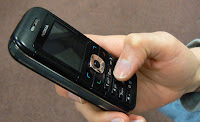## Tuesday, August 6, 2013

### The Origin of Jejenese (or, Jejenomics)Image from: journalism.co.uk

Just a thought.

The standard mobile phone keyboard contains the following character clusters:

A B C
D E F
G H I
J K L
M N O
P Q R S
T U V
W X Y Z

Reading from left to right, we assign a value of 1 on the leftmost character and increment it by 1 as we move to the right. We do this for each row and return to 1 when we start a new row.

Thus:

A = 1
B = 2
C = 3

D = 1
E = 2
F = 3
...

W = 1
X = 2
Y = 3
Z = 4

With me so far?

Good. Next.

Lets call the numbers "letter weights". A letter weight is the number of taps on a key you need to display a letter. Example: to type a lowercase 'c', we need to tap the 2 key three times.

To display a number 5 in a Nokia phone, you need to press the 5 key for about half a second. Knowing that, we assign a number weight = 1.5 for numbers.

Now, a "word weight" is the total of the letter weights in a word.

Example:

"dito" has a word weight of 9. That is (d=1) + (i=4) + (t=1) + (o=3)

Compare this to "d2" with a word weight of 2.5. (d=1) + (2=1.5)

The average texter will have their autospell feature turned off. In the absence of autospell, the goal of a frequent texter (eg, jejemon) is to conserve key-taps. This means using characters that have the lowest weights possible.

Let's test this theory on Jejenese words and compare them with normal spelling.

"jejeje": word weight = 9
"hehehe": word weight = 12

"eow p0h" is the Jejenese equivalent to the geek greeting "hello, world" (note that that's a zero between 'p' and 'h'). It has many variants in jejenese, but this most basic form represents a more jejenomic way of spelling an otherwise weighty "hello po". I'll leave it to you to do the math to compare its word weight with "hello po".

Why is the phrase terminated with an 'h' if the Jejenese really wanted to conserve? I have to theories on this anomaly.

One, the phrase used to be spelled "eow p0" but p0 might be read as p-zero, so the 'h' was added to emphasize that it's to be read as "po".

Two, after a while, Jejenese needed the equivalent of signature flourishes (or poetic license, if you may), so the 'h' was added as an embellishment.

Anyways, this is what happens to a bored and stressed out Silicon Carne.  :)

* * *
This post was quoted in a thesis written by Joseph Cataan at the UP College of Mass Communication. Link: Read at Scribd.